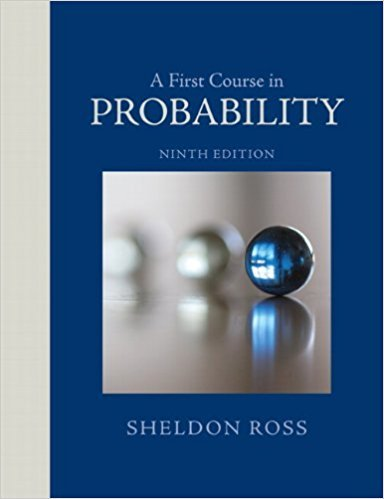×
×

# Solutions for Chapter 2: A First Course in Probability 9th Edition## Full solutions for A First Course in Probability | 9th Edition

ISBN: 9780321794772Solutions for Chapter 2

Solutions for Chapter 2
4 5 0 290 Reviews
16
5
##### ISBN: 9780321794772

Chapter 2 includes 97 full step-by-step solutions. This textbook survival guide was created for the textbook: A First Course in Probability , edition: 9. A First Course in Probability was written by and is associated to the ISBN: 9780321794772. Since 97 problems in chapter 2 have been answered, more than 62036 students have viewed full step-by-step solutions from this chapter. This expansive textbook survival guide covers the following chapters and their solutions.

Key Statistics Terms and definitions covered in this textbook
• `-error (or `-risk)

In hypothesis testing, an error incurred by rejecting a null hypothesis when it is actually true (also called a type I error).

• a-error (or a-risk)

In hypothesis testing, an error incurred by failing to reject a null hypothesis when it is actually false (also called a type II error).

• Attribute

A qualitative characteristic of an item or unit, usually arising in quality control. For example, classifying production units as defective or nondefective results in attributes data.

• Backward elimination

A method of variable selection in regression that begins with all of the candidate regressor variables in the model and eliminates the insigniicant regressors one at a time until only signiicant regressors remain

• Bimodal distribution.

A distribution with two modes

• Binomial random variable

A discrete random variable that equals the number of successes in a ixed number of Bernoulli trials.

• Causal variable

When y fx = ( ) and y is considered to be caused by x, x is sometimes called a causal variable

• Central composite design (CCD)

A second-order response surface design in k variables consisting of a two-level factorial, 2k axial runs, and one or more center points. The two-level factorial portion of a CCD can be a fractional factorial design when k is large. The CCD is the most widely used design for itting a second-order model.

• Confounding

When a factorial experiment is run in blocks and the blocks are too small to contain a complete replicate of the experiment, one can run a fraction of the replicate in each block, but this results in losing information on some effects. These effects are linked with or confounded with the blocks. In general, when two factors are varied such that their individual effects cannot be determined separately, their effects are said to be confounded.

• Conidence coeficient

The probability 1?a associated with a conidence interval expressing the probability that the stated interval will contain the true parameter value.

• Consistent estimator

An estimator that converges in probability to the true value of the estimated parameter as the sample size increases.

• Continuity correction.

A correction factor used to improve the approximation to binomial probabilities from a normal distribution.

• Cumulative normal distribution function

The cumulative distribution of the standard normal distribution, often denoted as ?( ) x and tabulated in Appendix Table II.

• Degrees of freedom.

The number of independent comparisons that can be made among the elements of a sample. The term is analogous to the number of degrees of freedom for an object in a dynamic system, which is the number of independent coordinates required to determine the motion of the object.

• Enumerative study

A study in which a sample from a population is used to make inference to the population. See Analytic study

• Error of estimation

The difference between an estimated value and the true value.

• False alarm

A signal from a control chart when no assignable causes are present

• Geometric random variable

A discrete random variable that is the number of Bernoulli trials until a success occurs.

• Goodness of fit

In general, the agreement of a set of observed values and a set of theoretical values that depend on some hypothesis. The term is often used in itting a theoretical distribution to a set of observations.

• Harmonic mean

The harmonic mean of a set of data values is the reciprocal of the arithmetic mean of the reciprocals of the data values; that is, h n x i n i = ? ? ? ? ? = ? ? 1 1 1 1 g .

×